Topics: Regression Analysis

Regression analysis can be a very powerful tool, which is why it is used in a wide variety of fields. The analysis captures everything from understanding the strength of plastic to the relationship between the salaries of employees and their gender. I've even used it for fantasy football! But there are assumptions your data must meet in order for the results to be valid. In this article, I'm going to focus on the assumptions that the error terms (or "residuals") have a mean of zero and constant variance.

When you run a regression analysis, the variance of the error terms must be constant, and they must have a mean of zero. If this isn't the case, your model may not be valid.

To check these assumptions, you should use a residuals versus fitted values plot. Below is the plot from the regression analysis I did for the fantasy football article mentioned above. The errors have constant variance, with the residuals scattered randomly around zero. If, for example, the residuals increase or decrease with the fitted values in a pattern, the errors may not have constant variance.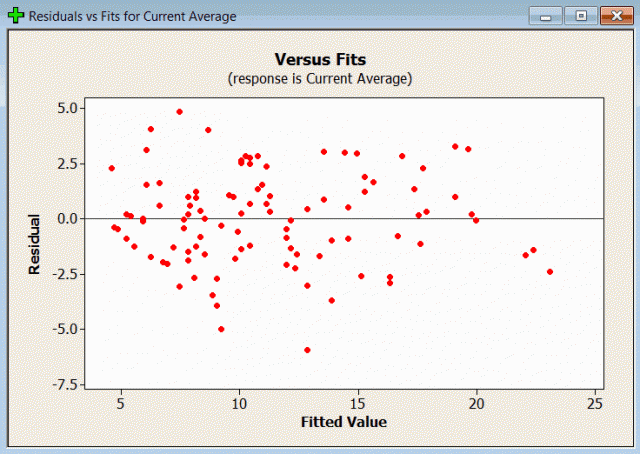The points on the plot above appear to be randomly scattered around zero, so assuming that the error terms have a mean of zero is reasonable. The vertical width of the scatter doesn't appear to increase or decrease across the fitted values, so we can assume that the variance in the error terms is constant.

But what if this wasn't the case? What if we did notice a pattern in the plot? I created some fake data to illustrate this point, then created another plot.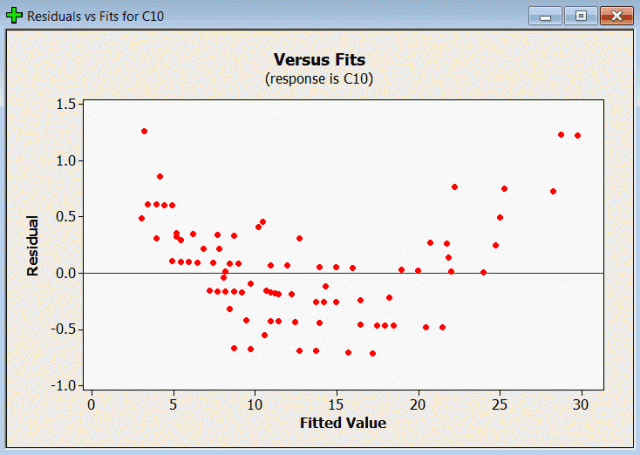There is definitely a noticeable pattern here! The residuals (error terms) take on positive values with small or large fitted values, and negative values in the middle. The width of the scatter seems consistent, but the points are not randomly scattered around the zero line from left to right.  This graph tells us we should not use the regression model that produced these results.

So what to do? There's no single answer, but there are several options. One approach is to adjust your model: adding a squared term to the model could solve the issue with the residuals plot.  Alternatively, Minitab has a tool that can adjust the data so that the model is appropriate and will yield acceptable residual plots. It's called a Box-Cox transformation, and it's easy to use! First just open the General Regression dialog (Stat > Regression > General Regression).  Then click the Box-Cox button.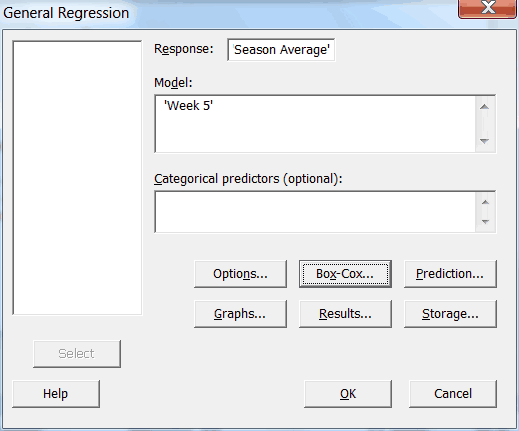The following dialog box will appear: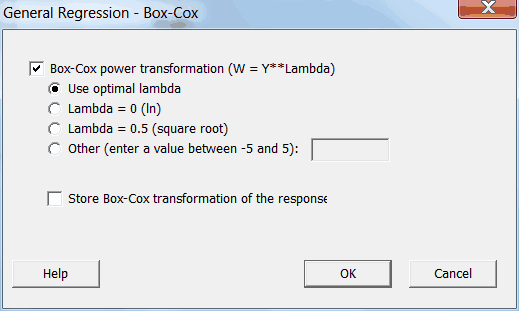Letting Minitab calculate the optimal lambda should produce the best-fitting results. That's what we'll do here.  After selecting the setting we want, just click "OK" and run the regression analysis as you normally would. Let's produce another plot to see if the transformation fixed the problem: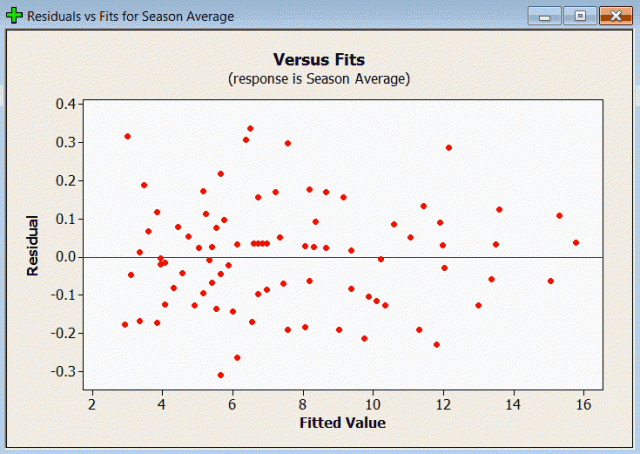And voila! No more patterns in the plot! Our assumption of constant variance and zero mean in the error terms has been met.